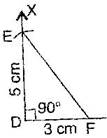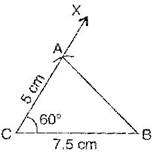### Practical Geometry - Solutions 3

CBSE Class –VII Mathematics
NCERT Solutions
Chapter 10 Practical Geometry (Ex. 10.3)

Question 1. Construct $\mathrm{\Delta }$DEF such that DE = 5 cm, DF = 3 cm and $m\mathrm{\angle }$EDF = ${90}^{\circ }.$
Answer: To construct$\mathrm{\Delta }$DEF where DE = 5 cm, DF = 3 cm and $m\mathrm{\angle }$EDF = ${90}^{\circ }.$Steps of construction:
(a) Draw a line segment DF = 3 cm.
(b) At point D, draw an angle of ${90}^{\circ }$ with the help of compass i.e., $\mathrm{\angle }$XDF = ${90}^{\circ }$.
(c) Taking D as centre, draw an arc of radius 5 cm, which cuts DX at the point E.
(d) Join EF.
It is the required right angled triangle DEF.
Question 2. Construct an isosceles triangle in which the lengths of each of its equal sides is 6.5 cm and the angle between them is ${110}^{\circ }.$
Answer: To construct: An isosceles triangle PQR where PQ = RQ = 6.5 cm and $\mathrm{\angle }$Q = ${110}^{\circ }.$
Steps of construction:
(a) Draw a line segment QR = 6.5 cm.
(b) At point Q, draw an angle of ${110}^{\circ }$ with the
help of protractor, i.e., $\mathrm{\angle }$PQR = ${110}^{\circ }.$
(d) Taking Q as centre, draw an arc with radius 1cm, which cuts QY at point P. 110${}^{\circ }$
(e) Join PR
It is the required isosceles triangle PQR.
Question 3. Construct $\mathrm{\Delta }$ABC with BC = 7.5 cm, AC = 5 cm and $m\mathrm{\angle }$C = ${60}^{\circ }.$
Answer: To construct$\mathrm{\Delta }$ABC where BC = 7.5 cm, AC = 5 cm and $m\mathrm{\angle }$C = ${60}^{\circ }.$Steps of construction:
(a) Draw a line segment BC = 7.5 cm.
(b) At point C, draw an angle of ${60}^{\circ }$ with the help of protractor, i.e., $\mathrm{\angle }$XCB = ${60}^{\circ }.$
(c) Taking C as centre and radius 5 cm, draw an arc, which cuts XC at the point A.
(d) Join AB
It is the required triangle ABC.